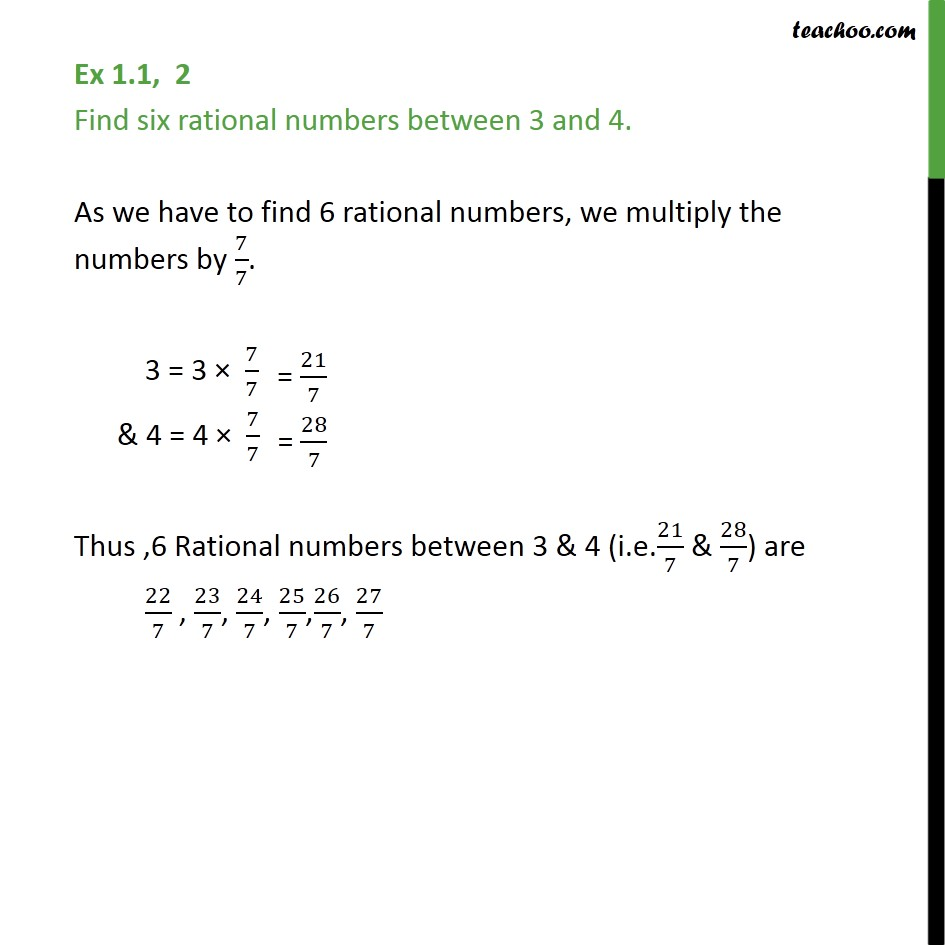1. Chapter 1 Class 9 Number Systems (Term 1)
2. Serial order wise
3. Ex 1.1

Transcript

Ex 1.1, 2 Find six rational numbers between 3 and 4. As we have to find 6 rational numbers, we multiply the numbers by 7/7. 3 = 3 7/7 & 4 = 4 7/7 Thus ,6 Rational numbers between 3 & 4 (i.e.21/7 & 28/7) are 22/7 , 23/7, 24/7, 25/7, 26/7, 27/7

Ex 1.1

Chapter 1 Class 9 Number Systems (Term 1)
Serial order wise